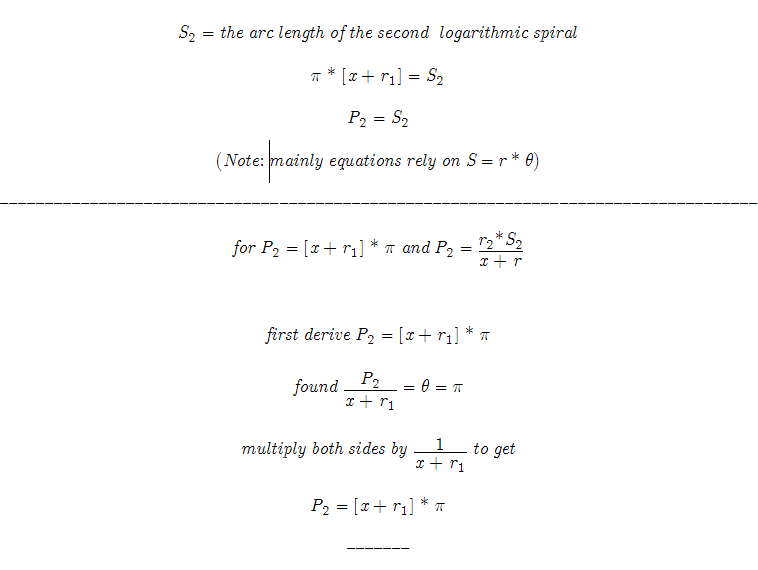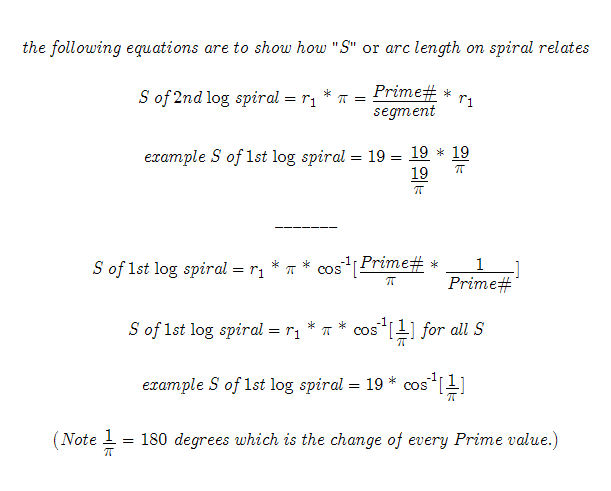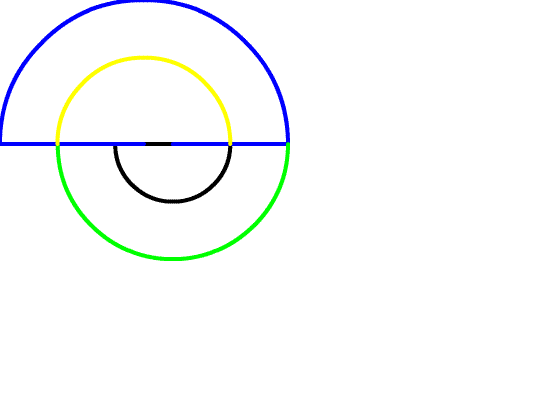_______ 20070618—20070619 It is important to read about the Prime number problem in this order: (Note all Math Hunches can be found here.) 1. This summary 2. Spiral Prime Numbers 3. Prime Number Theory 4. Prime# Solution 5. The sequence of Prime Codes The theory relies on the fact that there are 2 unique logarithmic spirals each of which have values based on a Prime number. And as the “arc” of the logarithmic spiral changes so does the values that it describes. Therefore the Prime numbers are revealed. The equations that describe logarithmic spirals can be difficult to use when trying to isolate values of single variables. That is why the equations used to find the logarithmic spirals use a well known Trigonometry equation: arc length equals radius times angle in radians or as commonly written: S = r * theta . So most all the equations that appear in this writeup use S = r * theta or are derived from that simple Trigonometry equation.Above Logarithmic Spiral has segment = (radius of Prime#) / Pi and each arc of the incircling spiral = to the Prime #The Above Logarithmic Spiral has a chord (the line shown under the arc) of (Prime number / Pi) Below is just an involute I was experimenting with.I will be adding more content to support this work soon. However do not wait and test the theory for yourself. May the Creative Force be with You!